Question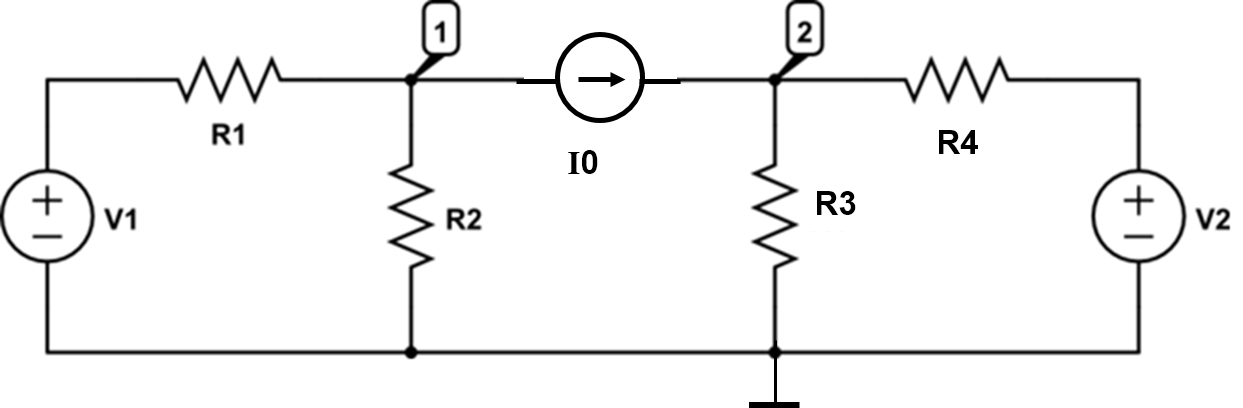In the circuit, V1=129 V, V2=72 V, I0=2 A, R1=7 Ohm, R2=46 Ohm, R3=19 Ohm, R4=19 Ohm.
Solve the circuit using nodal analysis and answer the questions below.

Find the current through R1 flowing in the direction from left to right. (Unit: A)

Find the current through R2 flowing downwards. (Unit: A)
Find the current through R3 flowing downwards. (Unit: A)
Find the current thrrough R4 flowing from left to right. (Unit: A)

We need at least 10 more requests to produce the answer.

0 / 10 have requested this problem solution

The more requests, the faster the answer.

All students who have requested the answer will be notified once they are available.

#### Earn Coins

Coins can be redeemed for fabulous gifts.

Similar Homework Help Questions
• ### Please show step by step solution and matlab code if possible. V R1 R4 R3 Q1....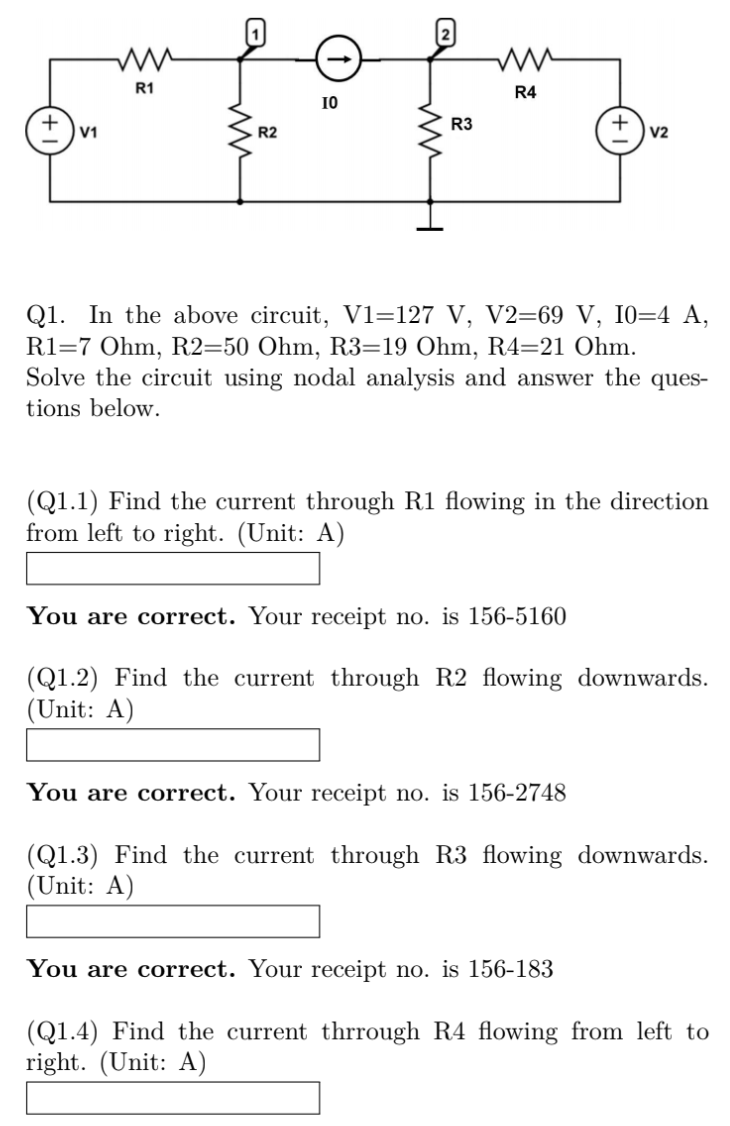Please show step by step solution and matlab code if possible. V R1 R4 R3 Q1. In the above circuit, V1=127 V, V2=69 V, 10=4 A, R1=7 Ohm, R2=50 Ohm, R3=19 Ohm, R4=21 Ohm. Solve the circuit using nodal analysis and answer the ques- tions below. (Q1.1) Find the current through R1 flowing in the direction from left to right. (Unit: A) You are correct. Your receipt no. is 156-5160 (Q1.2) Find the current through R2 flowing downwards. (Unit: A)...

• ### In the circuit, Vs1 = 10.5 V, Is2 = 0.45 A, R1 = 37 Ohm, R2...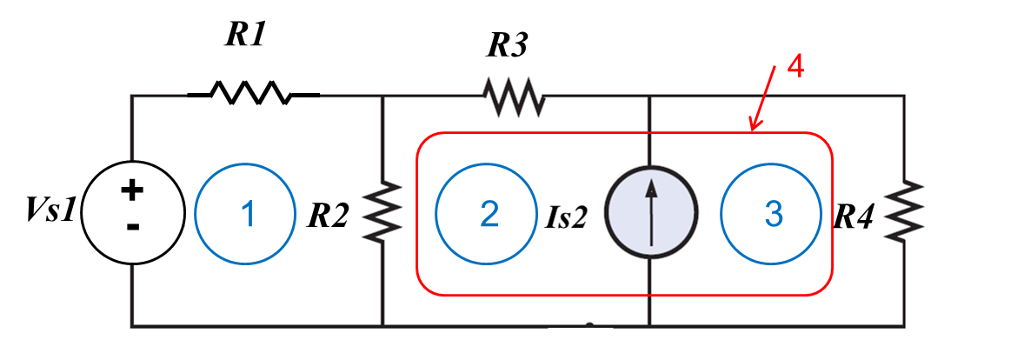In the circuit, Vs1 = 10.5 V, Is2 = 0.45 A, R1 = 37 Ohm, R2 = 19 Ohm, R3 = 36 Ohm, R4 = 26 Ohm. Solve the obtained equations and find the following currents. 1) Find the current through R1 flowing from left to right. (Unit: A) 2) Find the current through R2 flowing downwards. (Unit: A) 3) Find the current through R3 flowing from left to right. (Unit: A) RI R3 4 3

• ### Ri Vs S1 Q2. In the circuit, Vs 1 = 80 V, R1 = 4 Ohm,...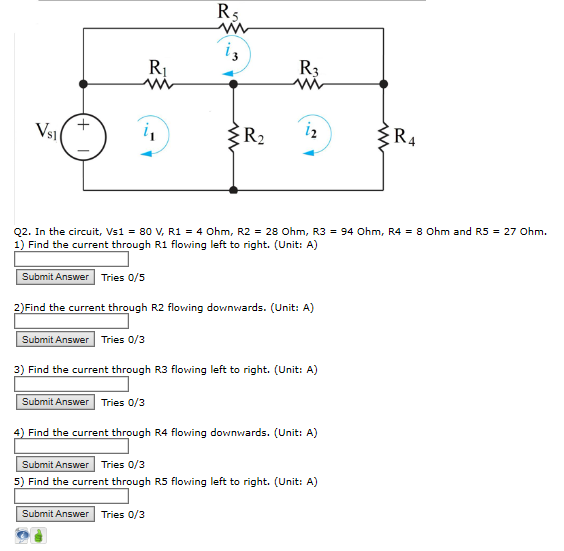Ri Vs S1 Q2. In the circuit, Vs 1 = 80 V, R1 = 4 Ohm, R2 = 28 Ohm, R3 = 94 Ohm, R4 = 8 Ohm and R5 = 27 Ohm. 1) Find the current through R1 flowing left to right. (Unit: A) Submit Answer Tries 0/5 2)Find the current through R2 flowing downwards. (Unit: A) Submit Answer Tries 0/3 3) Find the current through R3 flowing left to right. (Unit: A) Submit Answer Tries 0/3 4) Find...

• ### In the circuit shown, R1 = 7 ohm, R2 = 11 ohm, R3 = 9ohm, V1...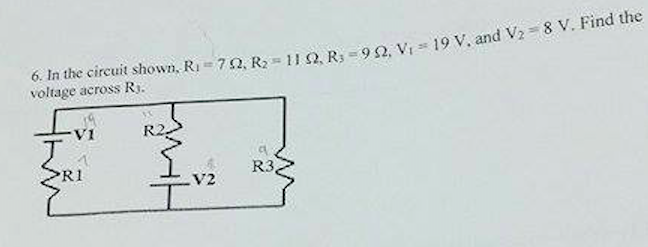In the circuit shown, R1 = 7 ohm, R2 = 11 ohm, R3 = 9ohm, V1 = 19 V, and V2 = 8V. Find the voltage across R3.

• ### R2 R3 R1 C1 C2 S2 51 In the circuit, the signal frequency f = 1.2 MHz, R1=9 Ohm, R2 = 8 Ohm, R3 =...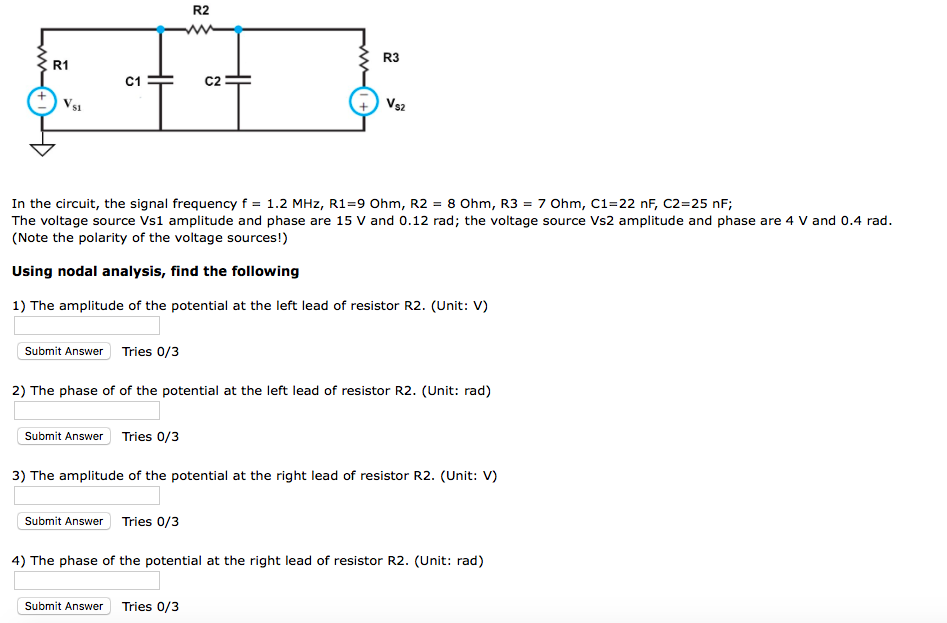PLEASE HELP IMMEDIATELY R2 R3 R1 C1 C2 S2 51 In the circuit, the signal frequency f = 1.2 MHz, R1=9 Ohm, R2 = 8 Ohm, R3 = 7 Ohm, C1-22 nF, C2=25 nF; The voltage source Vs1 amplitude and phase are 15 V and 0.12 rad; the voltage source Vs2 amplitude and phase are 4 V and 0.4 rad. (Note the polarity of the voltage sources!) Using nodal analysis, find the following 1) The amplitude of the potential at...

• ### Is1 2 R2 R1 Is2 R3 Vsl In the circuit, Vsi-24.8 V, Is1-2.1 A, 1s2-2.7 A,...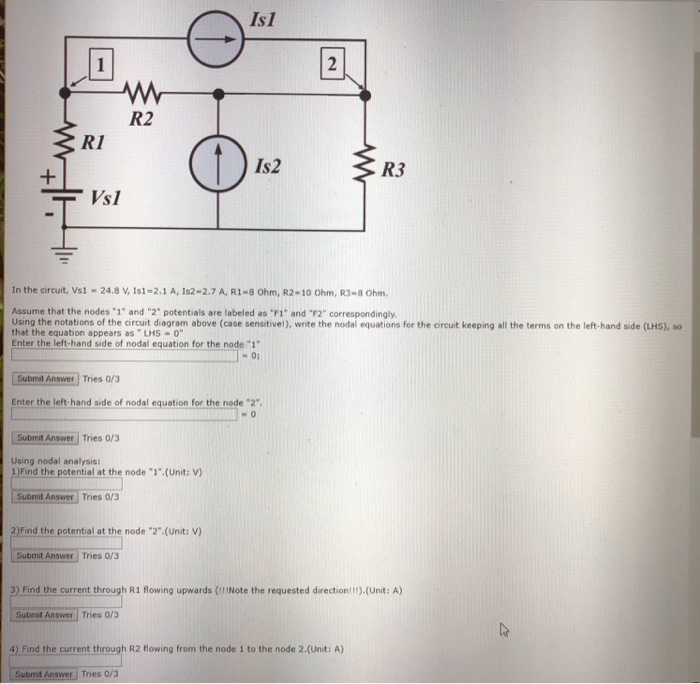Is1 2 R2 R1 Is2 R3 Vsl In the circuit, Vsi-24.8 V, Is1-2.1 A, 1s2-2.7 A, R1-8 Ohm, R2-10 Ohm, R3-8 Ohm Assume that the nodes-1" and "2" potentials are labeled as ℉1" and "F2" correspondingly. Using the notations of the ircuit diagram above (case sensitivel), wite the nodal equations for the Gircuit keeping all the terms on the left-hand side (LHS), so that the equation appears as LHS -0" Enter the left-hand side of nodal equation for the node...

• ### For the following circuit, assume that R1 = 1 ohm, R2 = 2 ohms, R3 =...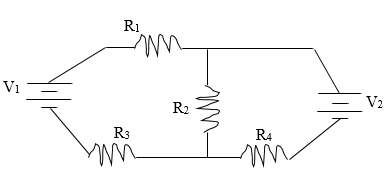For the following circuit, assume that R1 = 1 ohm, R2 = 2 ohms, R3 = 8 ohms, and R4 = 4 ohms. Also assume that V1 = 50 volts and V2 = 100 volts. Find the current through each of the resistors and the power dissipated through all of the resistors. (Hint: You’ll need to use Kirchoff’s Loop and Junction Rule) Vi R2 R3 R4 NV

• ### R1 13 |12 Vg V2 R3 R2 18 Ohm and R3 16 Ohm. 90 V, R2...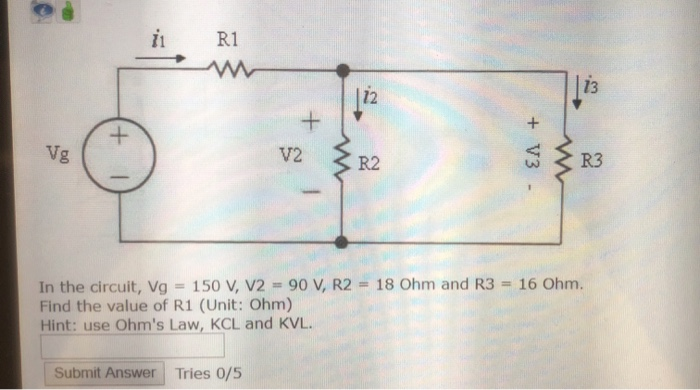R1 13 |12 Vg V2 R3 R2 18 Ohm and R3 16 Ohm. 90 V, R2 In the circuit, Vg Find the value of R1 (Unit: Ohm) Hint: use Ohm's Law, KCL and KVL 150 V, V2 Submit Answer Tries 0/5 +V3 +

• ### R2 R, Q2. In the above circuit, Vs1 = 11 V, Vs2 = 22 V, R1...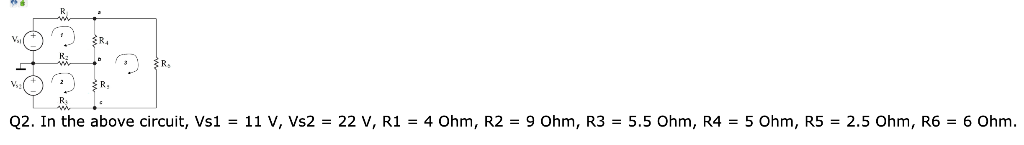R2 R, Q2. In the above circuit, Vs1 = 11 V, Vs2 = 22 V, R1 = 4 Ohm, R2 = 9 Ohm, R3 = 5.5 Ohm, R4 = 5 Ohm, R5 = 2.5 Ohm, R6 = 6 Ohm. B. Using mesh analysis, answer the following questions (Q2.2) Find the current through the resistor R1 in the direction from left to right. (Unit: A) Submit Answer Tries 0/3 (Q2.3) Find the current through the resistor R2 in the direction from...

• ### Find the node voltages v1, v, and v2. Use nodal analysis. R3 R 2 17 3...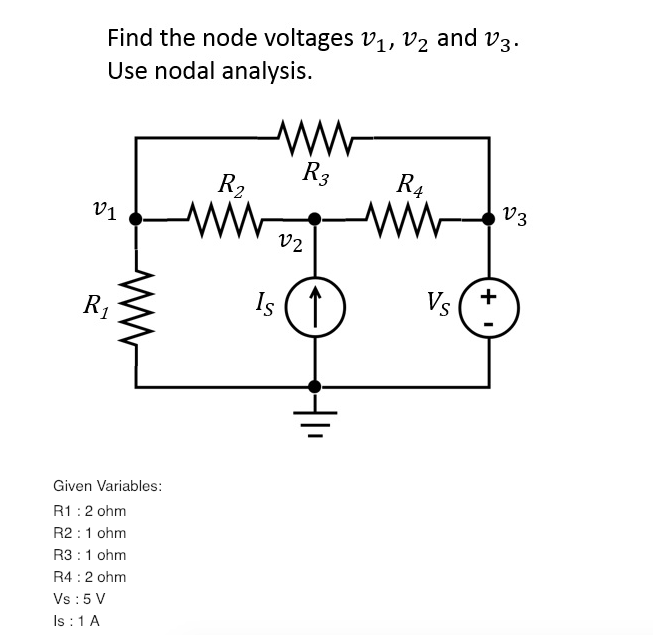Find the node voltages v1, v, and v2. Use nodal analysis. R3 R 2 17 3 1 2 Given Variables: R1:2 ohm R2 1 ohm R3 1 ohm R4 2 ohm Vs:5 V Is : 1 A